Mathematics D. Goguadze, P. Karchava Kolmogoroff's Extended Integral of the Abstract Set Many-Valued Complex-Value Function Presented by Member of the Academy L. Zhizhiashvili, December 9, 2003 ABSTRACT. In the present paper a notion of the normal class of the sets is introduced. In the terminology of the generalized multiplicative class a necessary and sufficient condition for the class of sets to be the normal class is established. Further Kolmogoroff's extended integral of the abstract set many-valued complex-value function is defined with respect to the normal class and, at the end, Fubini's generalized theorem is given. © 2004 Bull. Georg. Acad. Sci. Key words: normal class, directed set, many-valued complex-value set function, Kolmogoroff's extended integral. Mathematics T. AKHOBADZE ON GENERALIZED ÈESáRO SUMMABILITY OF TRIGONOMETRIC FOURIER SERIES Prezented by Member of the Academy L.Zhizhiashvili, November 26, 2003 ABSTRACT. The behavior of generalized Èesáro (C, an) means (anÎ(0,1], n=1,2,…) of trigonometric Fourier series of the class Hw in the space of continuous functions is studied. The obtained result is the best possible. © 2004 Bull. Georg. Acad. Sci. Key words: Èesáro (C, an) means, modulus of continuity. Mathematics D. LELADZE ON APPROXIMATE PROPERTIES OF CERTAIN SUBSEQUECES OF PARTIAL SUMS OF CONJUGATE SERIES OF MULTIPLE TRIGONOMETRIC FOURIER SERIES Presented by Member of the Academy L. Zhizhiashvili, December 16, 2003 ABSTRACT. In the present paper we give the estimate in the terms of partial and mixed moduli of continuity of deviation of multiple arithmetic means of the sequence of rectangular dyadedic partial sums of conjugate series of n-multiple trigonometric Fourier series of a function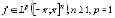or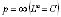, from n-multiple truncated conjugate function in the norm of . © 2004 Bull. Georg. Acad. Sci. Key words: trigonometric Fourier series, conjugate series. Mathematics T. KOPALIANI MAXIMAL OPERATOR IN GENERALIZED LEBESGUE SPACES WITH
 VARIABLE EXPONENT Presented by Member of the Academy L.Zhizhiashvili, October 21,2003 ABSTRACT. We give continuity conditions of the exponents p(x), q(x) which are sufficient for the Hardy-Littlewood maximal operator to be (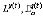) strong-type estimate. © 2004 Bull. Georg. Acad. Sci. Key words: variable exponent,Hardy-Littlewood maximal function,two-weighted estimate. Mathematics Z. Melikidze On the multiplicative complementation of some incomplete Haar type orthonormalized systems to bases in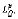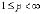Presented by Member of the Academy L. Zhizhiashvili, December 16, 2003 Abstract. In this paper we proved that if any finite collection of functions is removing from the Haar type system, there exists a bounded function such that after multiplying the remaining set of functions by this function the system obtained will be a basis in all spaces. For the Haar type system certain infinite removing is also possible. © 2004 Bull. Georg. Acad. Sci. Mathematics A. Lashkhi, P. GURTSKAIA, V. SESADZE CLASSICAL CONFIGURATIONS IN D-SEMIMODULAR LATTICES Presented by Corr. Member of the Academy A.Prangishvili, April 27, 2004 ABSTRACT. The idea to consider different unions of points, lines, planes, etc., is rather old. Many important configurations of such kinds are geometric (or matroidal) lattices. In this work we have studied configurations of Desargues, Pappus, Pasch in D-semimodular lattices. © 2004 Bull. Georg. Acad. Sci. Key words: geometry, semimodular lattice, collinear points. Mathematics T. Tsabadze On Similarity And Coordination Index Of Finite Collections Of Fuzzy Sets Presented by member of the Academy, May 3, 2004 ABSTRACT. This paper introduces theoretical basement of one approach to group decision-making in fuzzy enviroment. Mathematical apparatus, based on the concepts of coordination index and similarity of finite collections of fuzzy sets, has been successively constructed in metric lattice of fuzzy sets. © 2004 Bull. Georg. Acad. Sci. Key words: finite collection of fuzzy sets, regulation, coordination index, similarity. Mathematical Physics